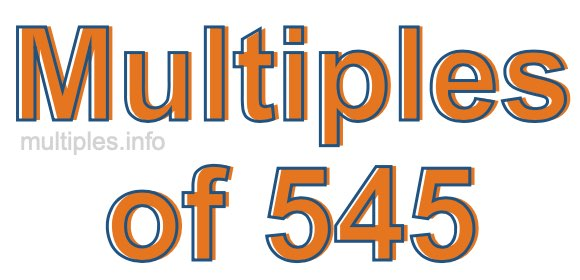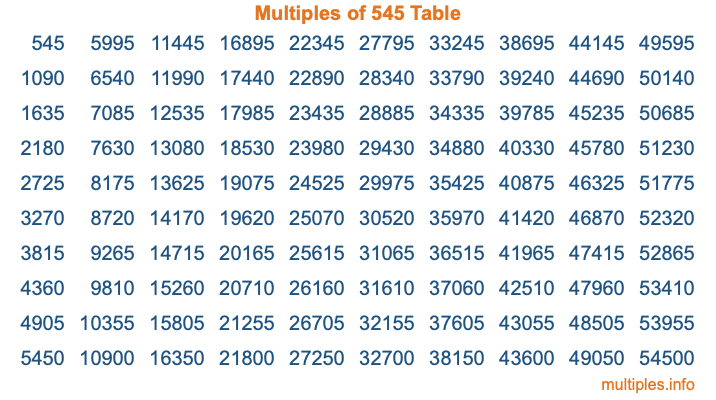Multiples of 545Welcome to the Multiples of 545 page. Here we will first teach you everything you will ever need to know about the multiples of 545, and then give you a study guide summary of everything we taught you to make sure you remember it all. Use this page to look up facts and learn information about the multiples of 545. This page will make you a multiples of five hundred forty-five expert!

Definition of Multiples of 545
Multiples of 545 are all the numbers that when divided by 545 equal an integer. Each of the multiples of 545 are called a multiple. A multiple of 545 is created by multiplying 545 by an integer.

Therefore, to create a list of multiples of 545, you start with 1 multiplied by 545, then 2 multiplied by 545, then 3 multiplied by 545, and so on for as long as you want. Thus, the list of the first five multiples of 545 is 545, 1090, 1635, 2180, and 2725. To see a larger list of multiples of 545, see the printable image of Multiples of 545 further down on this page. We also have a category where you can choose any nth multiple of 545.

Multiples of 545 Checker
The Multiples of 545 Checker below checks to see if any number of your choice is a multiple of 545. In other words, it checks to see if there is any number (integer) that when multiplied by 545 will equal your number. To do that, we divide your number by 545. If the the quotient is an integer, then your number is a multiple of 545.

Is  a multiple of 545?

Least Common Multiple of 545 and ...
A Least Common Multiple (LCM) is the lowest multiple that two or more numbers have in common. This is also called the smallest common multiple or lowest common multiple and is useful to know when you are adding our subtracting fractions. Enter one or more numbers below (545 is already entered) to find the LCM.

Check out our LCM Calculator if you need more details about the Least Common Multiple or if you need the LCM for different numbers for adding and subtraction fractions.

nth Multiple of 545
As we stated above, 545 is the first multiple of 545, 1090 is the second multiple of 545, 1635 is the third multiple of 545, and so on. Enter a number below to find the nth multiple of 545.

th multiple of 545

Multiples of 545 vs Factors of 545
545 is a multiple of 545 and a factor of 545, but that is where the similarities end. All postive multiples of 545 are 545 or greater than 545. All positive factors of 545 are 545 or less than 545.

Below is the beginning list of multiples of 545 and the factors of 545 so you can compare:

Multiples of 545: 545, 1090, 1635, 2180, 2725, etc.

Factors of 545: 1, 5, 109, 545

As you can see, the multiples of 545 are all the numbers that you can divide by 545 to get a whole number. The factors of 545, on the other hand, are all the whole numbers that you can multiply by another whole number to get 545.

It's also interesting to note that if a number (x) is a factor of 545, then 545 will also be a multiple of that number (x).

Multiples of 545 vs Divisors of 545
The divisors of 545 are all the integers that 545 can be divided by evenly. Below is a list of the divisors of 545.

Divisors of 545: 1, 5, 109, 545

The interesting thing to note here is that if you take any multiple of 545 and divide it by a divisor of 545, you will see that the quotient is an integer.

Multiples of 545 Table
Below is an image of the first 100 multiples of 545 in a table. The table is in chronological order, column by column. The first column has the first ten multiples of 545, the second column has the next ten multiples of 545, and so on.The Multiples of 545 Table is also referred to as the 545 Times Table or Times Table of 545. You are welcome to print out our table for your studies.

Negative Multiples of 545
Although not often discussed or needed in math, it is worth mentioning that you can make a list of negative multiples of 545 by multiplying 545 by -1, then by -2, then by -3, and so on, to get the following list of negative multiples of 545:

-545, -1090, -1635, -2180, -2725, etc.

Multiples of 545 Summary
Below is a summary of important Multiples of 545 facts that we have discussed on this page. To retain the knowledge on this page, we recommend that you read through the summary and explain to yourself or a study partner why they hold true.

There are an infinite number of multiples of 545.

A multiple of 545 divided by 545 will equal a whole number.

545 divided by a factor of 545 equals a divisor of 545.

The nth multiple of 545 is n times 545.

The largest factor of 545 is equal to the first positive multiple of 545.

545 is a multiple of every factor of 545.

545 is a multiple of 545.

A multiple of 545 divided by a divisor of 545 equals an integer.

545 divided by a divisor of 545 equals a factor of 545.

Any integer times 545 will equal a multiple of 545.

Multiples of a Number
Here you can get the multiples of another number, all with the same attention to detail as we did for multiples of 545 on this page.

Multiples of
Multiples of 546
Did you find our page about multiples of five hundred forty-five educational? Do you want more knowledge? Check out the multiples of the next number on our list!